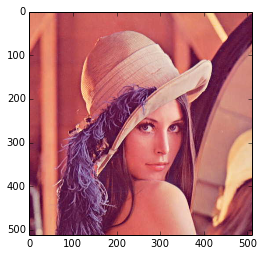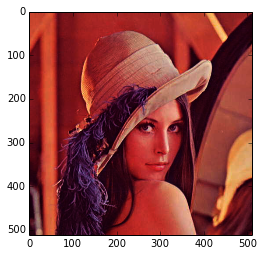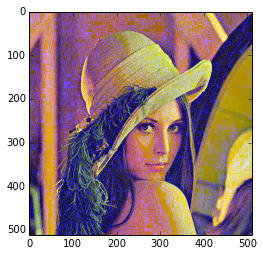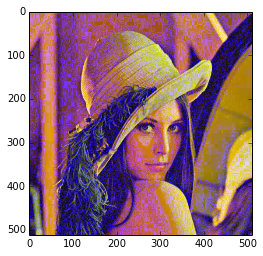In :
import numpy as np   # Standard numpy abbreviation : np
import mahotas as mh # Standard mahotas abbreviation : mh

In :
#im = mh.imread('lena.jpg') # loads lena image

(512, 512, 3)


im is just a numpy array, so you can use all numpy functionality with it.

This is one of the big advantages of the numpy ecosystem. In other languages, image libraries need to add functions for many things we get for free with numpy.

In :
print im.shape
r,g,b = im.transpose((2,0,1)) # Obtain the red/green/blue/channels with numpy methods

(512, 512, 3)

In :
imshow(im) # Display the image

Out:
<matplotlib.image.AxesImage at 0x4277b50>In :
# Let's play around with stretching the images:

print im ** 2.
imshow(im ** 2.)

[[[ 51984.  17956.  17424.]
[ 51984.  17956.  17424.]
[ 51984.  18225.  16900.]
...,
[ 59536.  22801.  18496.]
[ 51529.  17424.  12996.]
[ 38809.  10404.   6724.]]

[[ 51984.  18225.  16900.]
[ 51984.  18225.  16900.]
[ 51984.  18225.  16900.]
...,
[ 56169.  21025.  15376.]
[ 47961.  16129.  10404.]
[ 36481.  10000.   5329.]]

[[ 51529.  17956.  16641.]
[ 51529.  17956.  16641.]
[ 51529.  17956.  16129.]
...,
[ 55696.  21609.  13689.]
[ 46656.  16900.   9409.]
[ 36864.  11236.   5041.]]

...,
[[  7569.    484.   3136.]
[  7921.    576.   3364.]
[  8100.    625.   3481.]
...,
[ 31684.   4489.   5776.]
[ 32400.   4225.   5184.]
[ 32041.   3844.   4900.]]

[[  7569.    484.   3136.]
[  7744.    529.   3249.]
[  8100.    625.   3481.]
...,
[ 33489.   4624.   5625.]
[ 35344.   4489.   5184.]
[ 36100.   4489.   5184.]]

[[  7396.    441.   3025.]
[  7744.    529.   3249.]
[  8100.    625.   3481.]
...,
[ 34596.   4900.   5329.]
[ 37249.   4900.   5329.]
[ 38025.   5041.   5329.]]]

Out:
<matplotlib.image.AxesImage at 0x4291910>
<matplotlib.figure.Figure at 0x41ccc90>
In :
# Matplotlib does not deal with that image very well
# mahotas.stretch to the rescue: stretches the image to be in 0..255 range:
imshow(mh.stretch(im ** 2.))

Out:
<matplotlib.image.AxesImage at 0x48c5590>In :
# We can manipulate each channel independently and then use mh.as_rgb to put them back together:

imshow(mh.as_rgb(r**1.9, g, b**2))

Out:
<matplotlib.image.AxesImage at 0x48f6b10>In :
imshow(mh.as_rgb(r**1.9, g**1.2, b**2))

Out:
<matplotlib.image.AxesImage at 0x4c20050>In :
im_filtered = mh.as_rgb(r**1.9, g**1.2, b**2)
mh.imsave('lena_filtered.jpg', im_filtered)

In [ ]: